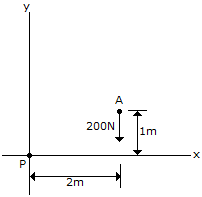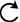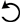# Engineering Mechanics - Force System Resultants - Discussion

### Discussion :: Force System Resultants - General Questions (Q.No.3)

3.Determine the magnitude and direction of the moment of the force of the movement if the force at A about point P.

 [A]. Mp = 400 Nm[B]. Mp = 400 Nm[C]. Mp = 200 Nm[D]. Mp = 200 NmExplanation:

No answer description available for this question.

 Swapnil Dafal said: (Oct 16, 2011) So simple moment =force * perpendicular distance between the two forces M=200*2 M=400 N-m (with respect to point P direction of moment is clockwise).

 Kusha Kumar.Poloju said: (Dec 26, 2012) Moment =force * perpendicular distance between the two forces M=200*2 M=400 N-m (With respect to point P direction of moment is clockwise).

 Kailas Jadhav said: (Jan 28, 2013) M=F*D (force into perpendicular distance). Hence moment about point p due to force 200n is M=+200*2=400(clockwise).

 Rajneeshkumar said: (Feb 5, 2013) M=F*D (force into perpendicular distance). Hence moment about point p due to force 200n is M=+200*2=400(clockwise).

 A.Kalyan Kumar said: (Jan 8, 2018) m=F*D(force in the perpendicular distance). Hence moment about point p due to force 200n is, M=200*2=400(clockwise).